# Understanding Word Embeddings Using Spacy Python

In this post, we will go over "What are Word Embeddings" and how to generate Word embeddings for stock tweets using Python package Spacy.

## Install Requirements

!pip install spacy

!spacy download en_core_web_lg

!pip install tweet-preprocessor

## Stock Tweets Data

Ok for this post, we will use stock tweets data. For data analyzing, we will use Python package pandas.

Let us look at our data first.

In :
import pandas as pd

In :
df = pd.read_csv("stocktweets/tweets/stocktwits.csv")

Out:
ticker message sentiment followers created_at
0 atvi $ATVI brutal selloff here today... really dumb... Bullish 14 2020-10-02T22:19:36.000Z 1 atvi$ATVI $80 around next week! Bullish 31 2020-10-02T21:50:19.000Z ## Cleaning the data We use tweet-preprocessor pip install tweet-preprocessor The following code will do... • Remove mentions and URLs • Remove non-alphanumeric characters • Rgnores Sentences with less than 3 words • Lower case everything • Remove redundant spaces In : import re import string import preprocessor as p from spacy.lang.en import stop_words as spacy_stopwords p.set_options(p.OPT.URL, p.OPT.MENTION) # removes mentions and URLs only stop_words = spacy_stopwords.STOP_WORDS punctuations = string.punctuation def clean(text): text = p.clean(text) text = re.sub(r'\W+', ' ', text) # remove non-alphanumeric characters # replace numbers with the word 'number' text = re.sub(r"\d+", "number", text) # don't consider sentenced with less than 3 words (i.e. assumed noise) if len(text.strip().split()) < 3: return None text = text.lower() # lower case everything return text.strip() # remove redundant spaces  Ok let us now remove the na using dropna() In : df = df.assign(clean_text=df.message.apply(clean)).dropna() df.head(2)  Out: ticker message sentiment followers created_at clean_text 0 atvi$ATVI brutal selloff here today... really dumb... Bullish 14 2020-10-02T22:19:36.000Z atvi brutal selloff here today really dumb giv...
1 atvi $ATVI$80 around next week! Bullish 31 2020-10-02T21:50:19.000Z atvi number around next week

## Spacy Word embeddings

In :
from IPython.display import Image
Image(filename="images/spacy_word_embeddings.png")

Out: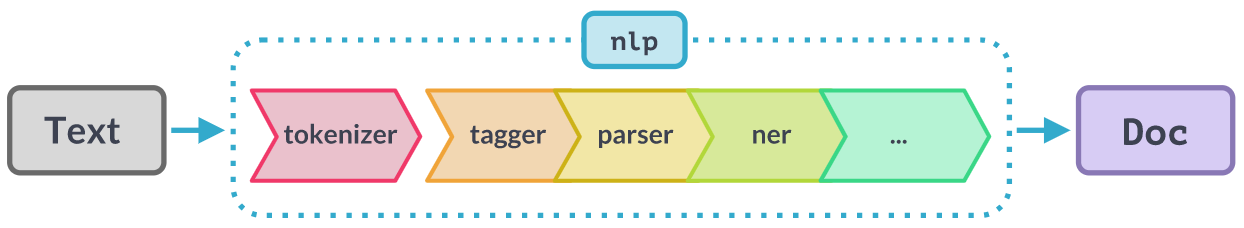In :
import spacy

In :
# for example
hello = nlp("hello")
hello.vector.shape # we get a 300-dimensional vector representing the word hello

Out:
(300,)

### Tokenization

Represent each sentence with its composing tokens.

In :
Image(filename="images/tokenization.png")

Out: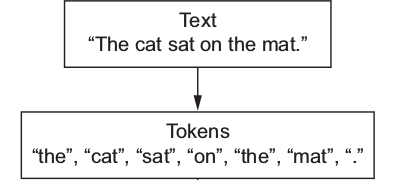Let us initialize our NLP tokenizer.

In :
# first we define our tokenizer
spacy_tokenizer = nlp.tokenizer
list(spacy_tokenizer("hello how are you"))

Out:
[hello, how, are, you]

### Lemmatization

We obtain the root of the words using lemmatization to have a cleaner and smaller set of vocabulary.

In :
Image(filename="images/lemmatization.png")

Out: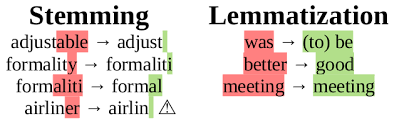For simplicity we will just assume that each tweet is one sentence. Below tokenize function does lemmatization and remove stop words.

In :
def tokenize(sentence):
sentence = nlp(sentence)
# lemmatizing
sentence = [ word.lemma_.lower().strip() if word.lemma_ != "-PRON-" else word.lower_ for word in sentence ]
# removing stop words
sentence = [ word for word in sentence if word not in stop_words and word not in punctuations ]
return sentence


Let us apply the tokenize function on a arbitrary sentence.

In :
tokenize("hello how are you this is a very interesting topic")

Out:
['hello', 'interesting', 'topic']

## Plot Word Embeddings

### Generate Vocab From Our Data

Let us import tqdm and initialize to keep track of our code(run) progress.

In :
from tqdm import tqdm
tqdm.pandas() # to keep track of our progress


Let us apply the tokenizer to our entire corpus first.

In :
sentences = df.clean_text.progress_apply(tokenize) # first we get list of lists of tokens composing each sentence
# this process takes a while!

100%|██████████| 29454/29454 [02:41<00:00, 182.49it/s]

In :
vocab = set()
for s in sentences:
vocab.update(set(s))

In :
vocab = list(vocab) # to make sure order matters

In :
print(f"We have {len(vocab)} tokens in our vocab")

We have 17066 tokens in our vocab


### Extracting The Vector for each token in Our Vocab

In :
# this also takes a while, but it is slightly faster than tokenization
vectors=[]
for token in tqdm(vocab):
vectors.append(nlp(token).vector)

100%|██████████| 17066/17066 [01:02<00:00, 272.11it/s]


### Projecting The Word Vectors onto a 2D Plane

We use PCA to reduce the 300 dimensions of our word embeddins into just 2 dimensions. If your data is 3D, then PCA tries to find the best 2D plane to capture most information from the data. In our case, the data is 300D, and we are looking for the best 2D plane to represent our data on. Each axis of the 2D plane we are trying to find is Principal Component (PC), hence the name Principal Component Analysis; the process of analyzing the data and finding the best principal components to represent the data with much smaller number of dimensions.

Example:

In :
Image(filename="images/pca.png")

Out: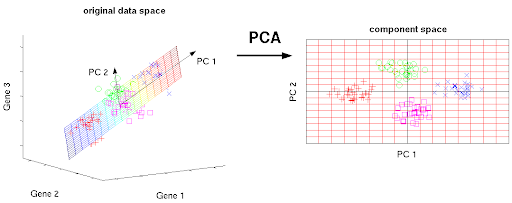### PCA Using Sklearn

In :
from sklearn.decomposition import PCA


The following code will transform our stock tweets data in to 2D data using sklearn principal component analysis.

In :
pca = PCA(n_components=2)
embeddings_2d = pca.fit_transform(vectors)


### Plotting the 2D Word Embeddings using Plotly

We will use plotly this time to be able to hover each embedding point and see which word it corresponds to!

I will use plolty to plot the word embeddings.

!pip install plotly

In [ ]:
import plotly.express as px
from plotly.offline import init_notebook_mode
init_notebook_mode() # required to reload the figures upon re-opening the notebook


Before we do plotting, we need to convert our word embedding vectors in to Pandas DataFrame.

In :
embeddings_df = pd.DataFrame({"x":embeddings_2d[:, 0], "y":embeddings_2d[:, 1], "token":vocab})


Below code will generate the scatter plot of our word embedding tokens.

In :
fig = px.scatter(embeddings_df, x='x', y='y', opacity=0.5, hover_data=['token'])
fig.show()

In :
Image(filename="images/embeddings_plot-min.png")

Out: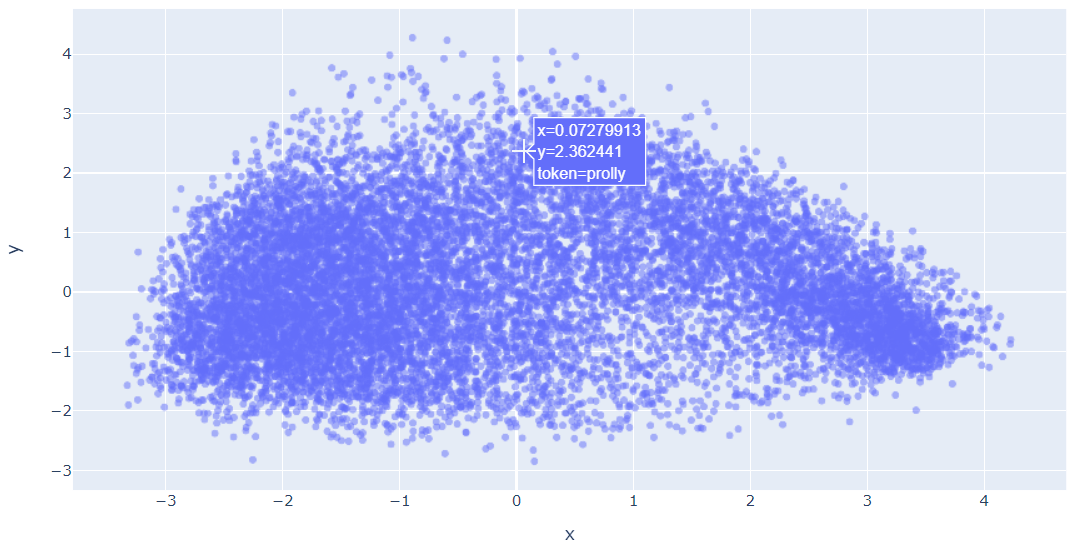Not showing the plot because of size.

### Plotting the 2D Word Embeddings using Matplotlib

In [ ]:
# you could also use matplotlib
import matplotlib.pyplot as plt

fig = plt.figure(figsize=(16, 9))

x_axis = embeddings_2d[:, 0]
y_axis = embeddings_2d[:, 1]

#plt.scatter(x_axis, y_axis, s=5, alpha=0.5) # alpha for transparency
#plt.show()


Not showing the plot because of size.

There we have it! Words represented numerically and even plotted on a 2D plane. Typically, if our dataset is sufficiently large, we can see words organized in a more meaningful way. We can even use these vectors to do word-math!

In :
Image(filename="images/word_embeddings_meaning.png")

Out: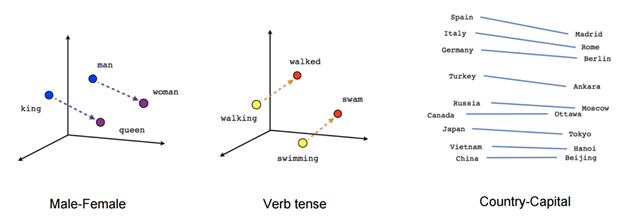Notice that we are using a pre-trained model from Spacy, that was trained on a different dataset. So even though our dataset is pretty small we can still represent our tweets numerically with meaningful embeddings, that is, similar tweets are going to have similar (or closer) vectors, and dissimilar tweets are going to have very different (or distant) vectors.

To check if we can use these embeddings to extract out any meaning from our stock tweets, we can use these as features in a downstream task, such as text classification.

## Text Classification using Word Embeddings

In :
Image(filename="images/text-classification-python-spacy.png")

Out: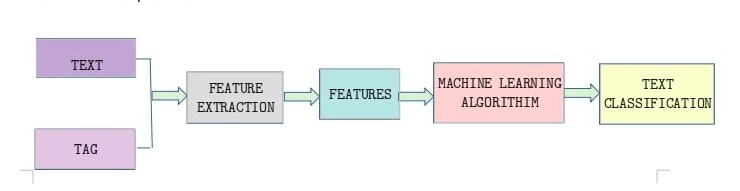### Use Sklearn to Generate Word Vectors from Sentences Automatically

Below code uses Sklearn's base class for transformers to fit and transform the data.

In :
# we just make a data type that has the functions fit and transform
from sklearn.base import TransformerMixin
class SpacyEmbeddings(TransformerMixin): # it inherits the sklearn's base class for transformers
def transform(self, X, **transform_params):
# Cleaning Text
return [sentence for sentence in X]

def fit(self, X, y=None, **fit_params):
return self

def get_params(self, deep=True):
return {}


### From Word Embeddings to Sentence Embeddings

We simply can take the sum of word embedding vectors, in what is called the Bag of Words (BOW) approach.

For example,

• v1 = [1, 2, 3]
• v2 = [3, 4, 5]
• v3 = [5, 6, 7]

Assume that the sentence that has the vectors v1, v2, and v3.Then the sentence vector will be...

sentence_vector = [9, 12, 15]

Count vectorizer from Sklearn can be used to generate the sentence vectors. Counter Vectorization uses bag-of-word.

Below code uses CountVectorizer with Spacy tokenizer.

In :
from sklearn.feature_extraction.text import CountVectorizer

bow_vector = CountVectorizer(tokenizer=spacy_tokenizer, ngram_range=(1,1))


We will go with something simple like a Decision Tree. Here is an example of a decision tree...

In :
Image(filename="images/Decision_Tree-2.png")

Out: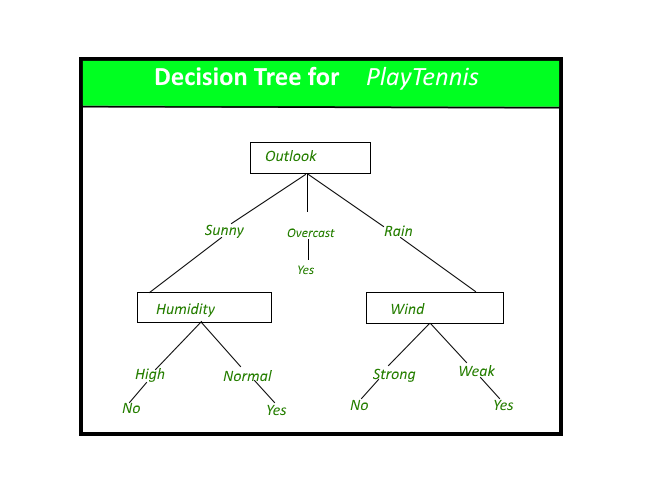The problem is, our dataset is very imbalanced. There are way more "Bullish" tweets than "Bearish" tweets. So we need to let the classifier know about this so it doesn't just classify everything as "Bullish".

#### Classify Stock Tweets using Sklearn Decision Tree Classifier

In :
from sklearn.tree import DecisionTreeClassifier
from sklearn.model_selection import train_test_split
from sklearn.utils.class_weight import compute_class_weight

In :
X, y = df["clean_text"], df["sentiment"]
# random_state ensures that whoever runs this notebook is going to get the same data split
X_train, X_test, y_train, y_test = train_test_split(X, y, random_state=42)

In :
class_weight = compute_class_weight(
class_weight='balanced', classes=["Bullish","Bearish"], y=y_train
)
class_weight

Out:
array([0.5926383 , 3.19866783])
In :
classifier = DecisionTreeClassifier(
class_weight={"Bullish":class_weight, "Bearish":class_weight}
)


#### Putting it all together

Ok, let us build the model using Sklearn pipeline. The input to our pipeline will be "word embeddings", "vectorizer" and then a "classifier" in the same order.

In :
from sklearn.pipeline import Pipeline # we use sklearn's pipeline

In :
# Create pipeline using Bag of Words
pipe = Pipeline([("embedder", SpacyEmbeddings()),
('vectorizer', bow_vector),
('classifier', classifier)])

pipe.fit(X_train, y_train)

Out:
Pipeline(steps=[('embedder',
<__main__.SpacyEmbeddings object at 0x7fdeb0cb7550>),
('vectorizer',
CountVectorizer(tokenizer=<spacy.tokenizer.Tokenizer object at 0x7fded6975f78>)),
('classifier',
DecisionTreeClassifier(class_weight={'Bearish': 3.198667825079641,
'Bullish': 0.5926383001556045}))])

### Evaluating the Word Embeddings based Classifier

To evaluate the model, let us try using our classifer to predict sentiment on our test data.

In :
predictions = pipe.predict(X_test)


Let us print our classification results.

In :
from sklearn.metrics import classification_report

In :
print(classification_report(y_test, predictions))

              precision    recall  f1-score   support

Bearish       0.00      0.00      0.00      1148
Bullish       0.84      1.00      0.92      6216

accuracy                           0.84      7364
macro avg       0.42      0.50      0.46      7364
weighted avg       0.71      0.84      0.77      7364


/home/abhiphull/anaconda3/envs/condapy36/lib/python3.6/site-packages/sklearn/metrics/_classification.py:1221: UndefinedMetricWarning: Precision and F-score are ill-defined and being set to 0.0 in labels with no predicted samples. Use zero_division parameter to control this behavior.
_warn_prf(average, modifier, msg_start, len(result))


It seems the model is still tending to classify everyhing as Bullish, this could mean that we need a better classifier to detect the patterns in the tweets, especially that this is a very challenging task to address with a simple classifier as Decision Tree. Nevertheless, the embeddings have proven to be useful to be used in downstream tasks as a way to represent tweets.